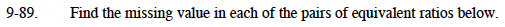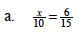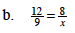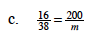### Home > MC1 > Chapter 9 > Lesson 9.2.4 > Problem9-89

9-89.If this were an equivalent fraction problem, how would you find the value of x?

Think about what values would go in a Giant One to turn tenths into fifteenths.

$\left(\frac{x}{10}\right)\left(\frac{1.5}{1.5}\right)=\frac{6}{15}$

This problem becomes x(1.5) = 6. What number multiplied by 1.5 gives 6?
You can also notice that 10 is two-thirds of 15 and think about what number is two-thirds of 6.

x = 4This problem is very similar to part (a).

x = 6How could you use a Giant One to find the value of m?